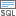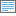# 条件函数 (Image Analyst)

## 使用情况

• 如果真栅格数据或可选假栅格数据为浮点型，则输出栅格数据也将为浮点型。 如果真表达式和可选假栅格数据均为整型，则输出栅格数据也将为整型。

• 如果输入条件栅格（Python 中的 in_conditional_raster）是单波段栅格，并且输入条件为真时所取的栅格数据或常量值（Python 中的 in_true_raster_or_constant）栅格或可选的输入条件为假时所取的栅格数据或常量值（Python 中的 in_false_raster_or_constant）栅格是常量，输出将是单波段栅格。

• 如果所有输入都是多波段栅格，则输出将是多波段栅格。 如果真输入或可选的假输入为常量，则输出栅格也将是多波段的。 每个多波段输入中的波段数必须相同。

• 该工具将使用来自其他输入的相应波段在条件栅格的每个波段上执行操作。 如果条件输入是多波段栅格，并且真或假栅格输入是常量，则该工具将使用多波段输入中每个波段的常量值执行操作。

• 如果表达式的评估结果非零，则将被视为 true。

• 如果未指定输入条件为假时所取的栅格数据或常量值，则将为表达式结果不为 true 的那些像元分配 NoData。

• 如果 NoData 不满足表达式，则像元不会接收输入条件为假时所取的栅格数据值；像元值仍是 NoData。

• 表达式使用 SQL 查询。 有关创建查询的详细信息，请参阅以下主题：

• 要在 Python 中使用 {where_clause}，应以引号括起来。 例如，"Value > 5000”

有关使用 Python 指定查询的详细信息，请参阅帮助。

• 在 Python 中，您可避免使用 {where_clause}，其通过将地图代数表达式用作 in_conditional_raster 来指定 Value 字段。

例如，以下表达式：

• Con("elev", 0, 1, "value > 1000")

可被重新编写，如下所示：

• Con(Raster("elev") > 1000, 0, 1)

有关详细信息，请参阅下列代码示例或在地图代数中查看构建复杂语句

• 逻辑表达式的最大长度为 4,096 个字符。

## 参数

 标注 说明 数据类型 输入条件栅格数据 表示所需条件结果为真或假的输入栅格。可以是整型或浮点型。 Raster Layer 输入条件为真时所取的栅格数据或常量值 条件为真时，其值作为输出像元值的输入。可为整型或浮点型栅格，或为常数值。 Raster Layer; Constant 输入条件为假时所取的栅格数据或常量值(可选) 条件为假时，其值作为输出像元值的输入。可为整型或浮点型栅格，或为常数值。 Raster Layer; Constant 表达式(可选) 决定输入像元为真或假的逻辑表达式。 Where 子句遵循 SQL 表达式的一般格式。 如果您单击编辑 SQL 模式按钮，则可以直接输入，例如 VALUE > 100。 如果处于编辑子句模式中，则可以通过单击添加子句模式按钮来开始构建表达式。 SQL Expression

### 返回值

 标注 说明 数据类型 输出栅格 输出栅格。 Raster

`Con(in_conditional_raster, in_true_raster_or_constant, {in_false_raster_or_constant}, {where_clause})`
 名称 说明 数据类型 in_conditional_raster 表示所需条件结果为真或假的输入栅格。可以是整型或浮点型。 Raster Layer in_true_raster_or_constant 条件为真时，其值作为输出像元值的输入。可为整型或浮点型栅格，或为常数值。 Raster Layer; Constant in_false_raster_or_constant(可选) 条件为假时，其值作为输出像元值的输入。可为整型或浮点型栅格，或为常数值。 Raster Layer; Constant where_clause(可选) 决定输入像元为真或假的逻辑表达式。表达式遵循 SQL 表达式的一般格式。 where_clause 的示例为 "VALUE > 100"。 SQL Expression

### 返回值

 名称 说明 数据类型 out_raster 输出栅格。 Raster

### 代码示例

Con 示例 1（Python 窗口）

``````import arcpy
from arcpy import env
from arcpy.ia import *
env.workspace = "C:/output/data"
outCon = Con("elevation", "elevation", "", "VALUE > 2000")
outCon.save("C:/output/outcon.img")

# Execute Con using a map algebra expression instead of a where clause
outCon2 = Con(Raster("elevation") > 2000, "elevation")
outCon2.save("C:/output/outcon2")``````
Con 示例 2（Python 窗口）

``````# Name: Con_Ex_02.py
# Description: Performs a conditional if/else evaluation
#              on each pixel of an input raster.
# Requirements: Image Analyst Extension

# Import system modules
import arcpy
from arcpy import env
from arcpy.ia import *

# Set environment settings
env.workspace = "C:/output"

# Set local variables
inRaster = Raster("elevation")
inTrueRaster = 1
inFalseConstant = 0
whereClause = "VALUE >= 1500"

# Check out the ArcGIS Image Analyst extension license
arcpy.CheckOutExtension("ImageAnalyst")

# Execute Con and save the output
outCon = Con(inRaster, inTrueRaster, inFalseConstant, whereClause)
outCon.save("C:/output/outcon.tif")

# Execute Con using a map algebra expression instead of a where clause, and save the output.
outCon2 = Con(inRaster >= 1500, inTrueRaster, inFalseConstant)
outCon2.save("C:/output/outcon2.tif")``````

## 许可信息

• Basic: 需要 Image Analyst 或 Spatial Analyst
• Standard: 需要 Image Analyst 或 Spatial Analyst
• Advanced: 需要 Image Analyst 或 Spatial Analyst